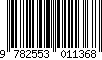# Solved Problems in Electrochemistry for Universities and Industry

## Auteur : PIRON Dominique L.

Langue : Anglais## Thèmes de Solved Problems in Electrochemistry for Universities and... :

Date de parution :
Ouvrage 220 p. · 17x25 cm · Broché
ISBN : 9782553011368Presses internationales Polytechnique

• ## Sommaire

This book was written for two groups: (1) professors and students in chemistry, materials, metallurgy and chemical engineering, and (2) practicing engineers and technicians working in corrosion, electrolysis, plating, electrochemical cells and rechargeable batteries. This book fills the need for a modern approach to problem solving and calculations in electrochemistry. It provides a useful text that is not currently available. The examples of solutions of specific cases presented here benefit students and professors and also represent a useful tool for scientists, engineers and technicians working in electrochemistry. This approach develops the aptitude for obtaining numerical values as answers to specific questions, which is an essential requirement for the design and control of industrial processes and for laboratory experiments. This book presents calculated solutions to problems in fundamental and applied electrochemistry. It uses industrial data to illustrate scientific concepts and scientific knowledge to solve practical problems. It is subdivided into three parts. The first uses modern basic concepts, the second studies the scientific basis for electrode and electrolyte thermodynamics (including E-pH diagrams and the minimum energy involved in transformations) and the kinetics of rate processes (including the energy lost in heat and in parasite reactions). The third part treats larger problems in electrolysis and power generation, as well as in corrosion and its prevention. Each chapter includes three sections: (1) the presentation of useful principles, (2) some twenty problems with their solutions, and (3) a set of unsolved problems.
USEFUL BASIC ELECTROCHEMISTRY. Chapter 1. Use of Electrode Concepts. Chapter 2. Faraday's Law. FUNDAMENTAL SCIENCE AND ITS PRACTICAL USE. Chapter 3. Electrode thermodynamics and E-pH diagrams. Chapter 4. Electrolyte Solutions. Chapter 5. Electrode Kinetics (Processes). INDUSTRIAL PROBLEMS (MORE COMPLEX PROBLEMS, USING ASPECTS OF SEVERAL PREVIOUS CHAPTERS). Chapter 6. Electrolysis production problems. Chapter 7. Corrosion Failure and Prevention Problems. Chapter 8. Electrochemical power generators.